# Plus One Chemistry Notes Chapter 1 Some Basic Concepts of Chemistry

﻿Students can Download Chapter 1 Some Basic Concepts of Chemistry Notes, Plus One Chemistry Notes helps you to revise the complete Kerala State Syllabus and score more marks in your examinations.

## Kerala Plus One Chemistry Notes Chapter 1 Some Basic Concepts of Chemistry

INTRODUCTION
Chemistry is the branch of science which deals with the composition, properties and transformation of matter. These aspects can be best understood in terms of basic constituents of matter: atoms and molecules. That is why chemistry is called the sci-ence of atoms and molecules.

IMPORTANCE OF CHEMISTRY
Chemistry plays an important role in almost all walks of life. In recent years chemistry has tackled with a fair degree of success some of the pressing aspects of environmental degradation. Safer alternatives to environmentally hazardous refrigerants like CFCs, responsible for ozone depletion in the stratosphere, have been successfully synthesised.

NATURE OF MATTER
Matter is anything which has mass and occupies space. Matter can exist in three physical states: solid, liquid and gas.

At the macroscopic or bulk level, matter can be clas-sified as mixtures or pure substances.
A material containing only one substance is called a pure substance. Materials containing more than one substance are called mixtures. Pure substances are further classified into two types: elements and com¬pounds. Mixtures are also of two types: homoge¬neous mixtures and heterogeneous mixtures. A mix¬ture is said to be homogeneous if it has same com¬position throughout. Some examples of homoge¬neous mixtures are air, gasoline, kerosene, milk, alloys, etc. Heterogeneous mixtures are the mixtures which have different composition in different parts. Some examples of heterogeneous mixtures are iron and sulphur, muddy water, etc.

PROPERTIES OF MATTER AND THEIR MEASUREMENT
Every substances has characteristic properties. These are classified into two categories – physical properties and chemical properties.
Physical properties are those properties which can be measured or observed without changing the iden¬tity or the composition of the substance. Some ex¬amples of physical properties are colour, odour, melt¬ing point, boiling point, density, etc. chemical properties are characteristic reactions of different substances; these include acidity or basic¬ity, combustibility, etc.

THE INTERNATIONAL SYSTEM OF UNITS (SI UNITS)
A unit may be defined as the standard of reference chosen to measure any physical quantity. There are many different systems of units.
The improved metric system of units accepted inter-nationally is called International System of Units or SI units. The SI system has seven base units from which all other units are derived.

UNCERTAINTY IN MEASUREMENT
Scientific measurements involving some measuring devices have some degree of uncertainty. The magnitude of uncertainty depends on the accuracy of the measuring device and also on the skill of its operator.
The closeness of a set of values obtained from identical measurements of a quantity is known as precision of the measurement.
The term accuracy is defined as the closeness of a measurement or a set of measurements to its true value.

SIGNIFICANT FIGURES
The total number of digits in a measurement is called the number of significant figures. It includes the num¬ber of figures that are known with certainty plus the last uncertain digit, beginning with the first non-zero digit.
1) All non-zero digits are significant. For example, in 285 cm, there are three significant figures and in 0.25 mL, there are two significant figures.2) Zeros preceding to first non-zero digit are not significant. Such zero indicates the position of decimal point. Thus, 0.03 has one significant figure and 0.0052 has two significant figures.
3) Zeros between two non-zero digits are significant. Thus, 2.005 has four significant figures.

4) Zeros at the end or right of a number are significant provided they are on the right side of the decimal point. For example,0.200 g has three significant figures. But, if otherwise, the zeros are not significant. For example, 100 has only one significant figure.

5) Exact numbers have an infinite number of significant figures. For example, in 2 balls or 20 eggs, there are infinite significant figures as these are exact numbers and can be represented by writing infinite number of zeros after placing a decimal i.e.,2 = 2.000000 or 20 = 20.000000

LAWS OF CHEMICAL COMBINATIONS
Law of Conservation of Mass
It states that matter can neither be created nor destroyed.
This law was put forth by Antoine Lavoisier in 1789.

Law of Definite Proportions
This law was given by, a French chemist, Joseph Proust. He stated that a given compound always contains exactly the same proportion of elements by weight. It is sometimes also referred to as Law of definite composition.Law of Multiple Proportions
This law was proposed by Dalton in 1803. According to this law, if two elements can combine to form more than one compound, the masses of one element that combine with a fixed mass of the other element, are in the ratio of small whole numbers.

Gay Lussac’s Law of Gaseous Volumes
This law was given by Gay Lussac in 1808. He observed that when gases combine or are produced in a chemical reaction they do so in a simple ratio by volume provided all gases are at same temperature and pressure.

In 1811, Avogadro proposed that equal volumes of gases at the same temperature and pressure should contain equal number of molecules Avogadro made a distinction between atoms and molecules which is quite understandable in the present times.

DALTON’S ATOMIC THEORY
The main postulates of the theory are:-

1. Matter is made up of extremely small, indivisible particles called atoms.
2. Atoms of the same element are identical in all respects i.e. size and mass.
3. Atoms of different elements are different, i.e., they possess different sizes, shapes, masses, and chemical properties.
4. Atoms of different elements may combine with each other in a simple whole number ratio to form compound atoms or molecules.
5. Atoms can neither be created nor destroyed, i.e., atoms are indestructible.

ATOMIC AND MOLECULAR MASSES
ATOMIC MASS
The mass of an atom is extremely small. These are very inconvenient for calculations. This difficulty was overcome by expressing atomic masses as relative masses, i.e., with respect to the mass of an atom of a standard substance. The scale in which the relative masses are expressed is called atomic mass unit scale or amu scale. One atomic mass unit (amu) is equal to one-twelfth (1/ 12) the mass of an atom of carbon-12. Recently the symbol ‘u’ (unified mass) is used in place of amu.AVERAGE ATOMIC MASS
The atomic masses of many elements have fractional values because they exist as mixture of isotopes.
In the case of such elements, the atomic mass is taken as the average of the atomic masses of the various isotopes. For example, ordinary chlorine is a mixture of two isotopes with atomic masses 35 u and 37 u and they are present in the ration 3:1. Therefore,
Atomic mass of Chlorine = $$\frac{35 \times 3+37 \times 1}{3+1}$$ = 35.5uMOLECULAR MASS
Molecular mass is the sum of atomic masses of the elements present in a molecule.
For example, molecular mass of water
= 2 x atomic mass of hydrogen + 1 x atomic mass of oxygen
= 2 × (1.008u) + 1 × 16.00 u
= 18.02 u

FORMULA MASS
In ionic compounds, we use formula mass instead of molecular mass. Formula mass of an ionic compound is the sum of the atomic masses of all atoms in a formula unit of the compound.

MOLE CONCEPT AND MOLAR MASSES
‘Mole’ was introduced as the seventh base quantity for the amount of substance in SI system. One mole is the amount of a substance that contains as many particles or entities as there are atoms in exactly 12 g (or 0.012 kg) of the 12C isotope. This number is known as ‘Avogadro constant’ (NA = 6.022 × 1023). The mass of one mole of a substance in grams is called its molar mass. The molar mass is numerically equal to atomic/ molecular/ formula mass in u.

PERCENTAGE COMPOSITION
One can check the purity of a sample by analysing its mass percentage
Mass % of an element in a compound =EMPIRICAL FORMULA FOR MOLECULAR FORMULA
An empirical formula represents the simplest whole number ratio of various atoms present in a compound whereas the molecular formula shows the exact number of different types of atoms present in a molecule of a compound.

Problem 1.2
A compound contains 4.07 % hydrogen, 24.27 % carbon and 71.65 % chlorine. Its molar mass is 98.96 g. What are its empirical and molecular formulae?
Solution:
Step 1. Conversion of mass per cent to grams.
Since we are having mass per cent, it is convenient to use 100 g of the compound as the starting material. Thus, in the 100 g sample of the above compound, 4.07g hydrogen is present, 24.27g carbon is present and 71.65 g chlorine is present.

Step 2. Convert into number moles of each element
Divide the masses obtained above by respective atomic masses of various elements.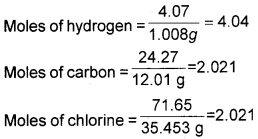Step 3. Divide the mole value obtained above by the smallest number
Since 2.021 is smallest value, division by it gives a ratio of 2:1:1 for H:C:CI.
In case the ratios are not whole numbers, then they may be converted into whole number by multiplying by the suitable coefficient.

Step 4. Write empirical formula by mentioning the numbers after writing the symbols of respective elements.
CH2Cl is, thus, the empirical formula of the above compound.

Step 5. Writing molecular formula
a) Determine empirical formula mass. Add the atomic masses of various atoms present in the empirical formula.
ForCH2Cl, empirical formula mass is 12.01 + 2 1.008 + 35.453 = 49.48 g

b) Divide Molar mass by empirical formula massc) Multiply empirical formula by ‘n’ obtained above to get the molecular formula
Empirical formula = CH2Cl, n = 2.
Molecular formula = 2 × [CH2Cl]=C2H4Cl2

STOICHIOMETRY AND STOICHIOMETRIC CALCULATIONS
‘Stoichiometry’ deals with the calculation of masses (sometimes volumes also) of the reactants and prod¬ucts involved in a chemical reaction. The coefficients of reactants and products in a balanced chemical equation is called the stoichiometric coefficients.

LIMITING REAGENT
The amount of the product obtained in a chemical reaction is determined by the amount of the reactant that is completely consumed in the reaction. This reactant is called the limiting reagent. Thus, limiting reagent may be defined as the reactant which is com¬pletely consumed in a reaction containing two or more reactants.

REACTIONS IN SOLUTIONS
1. Mass per cent :
It is obtained by the following relation: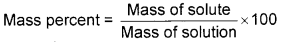2.Mole Fraction :
It is the ratio of number of moles of a particular component to the total number of moles of the solution. If a substance ‘A’ dissolves in substance ‘B’ and their number of moles are nA and nB respectively; then the mole fractions of A and B are given as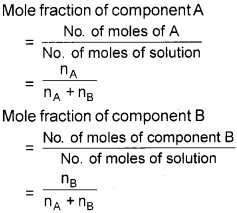3. Molarity :
It is the most widely used unit and is denoted by M. It is defined as the number of moles of the solute in 1 litre of the solution.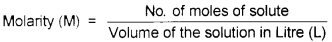4. Molality :
It is defined as the number of moles of solute present in 1 kg of solvent. It is denoted by ‘m’.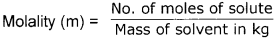Note :
Molarity of a solution changes with temeprature. But molality of a solution does not change with temperature since mass remains unaffected with temperature.